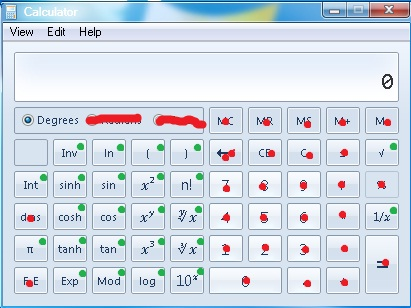# Calculator problem

Algebra Level 2Note: This problem is incorrect.

I want to calculate the square root of 7 correct to at least 5 decimal places. However, my calculator is dysfunctional and only the green keys work (the red keys do not).

How many key presses are required to display the square root of 7 starting from the All Clear (0) position.

For example, if I wanted to get $0.1$, then starting from 0, pressing $10^x$ will give me 1. Pressing $10^x$ will give me $10$. Pressing $1/x$ will give me $0.1$.

Edit: Sorry. Somebody has pointed out that it can be done in 4 steps using Factorial of Pi. He is right. I was under the impression that the factorial function was defined only for non-negative integers.
Someone else has also pointed a way of doing this with fewer than 9 steps, so the options are not correct.

Anyway, for those still interested, try finding the minimum number of steps needed.

×